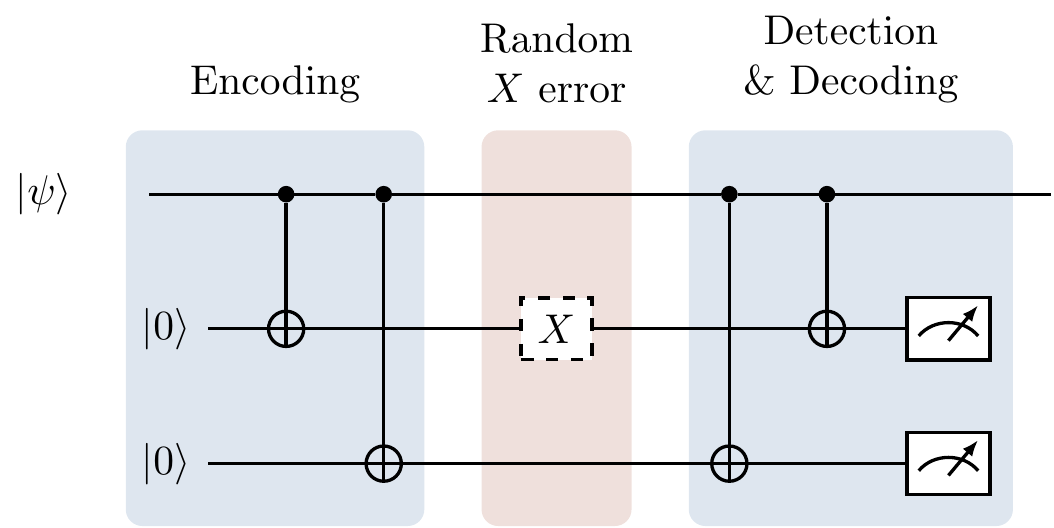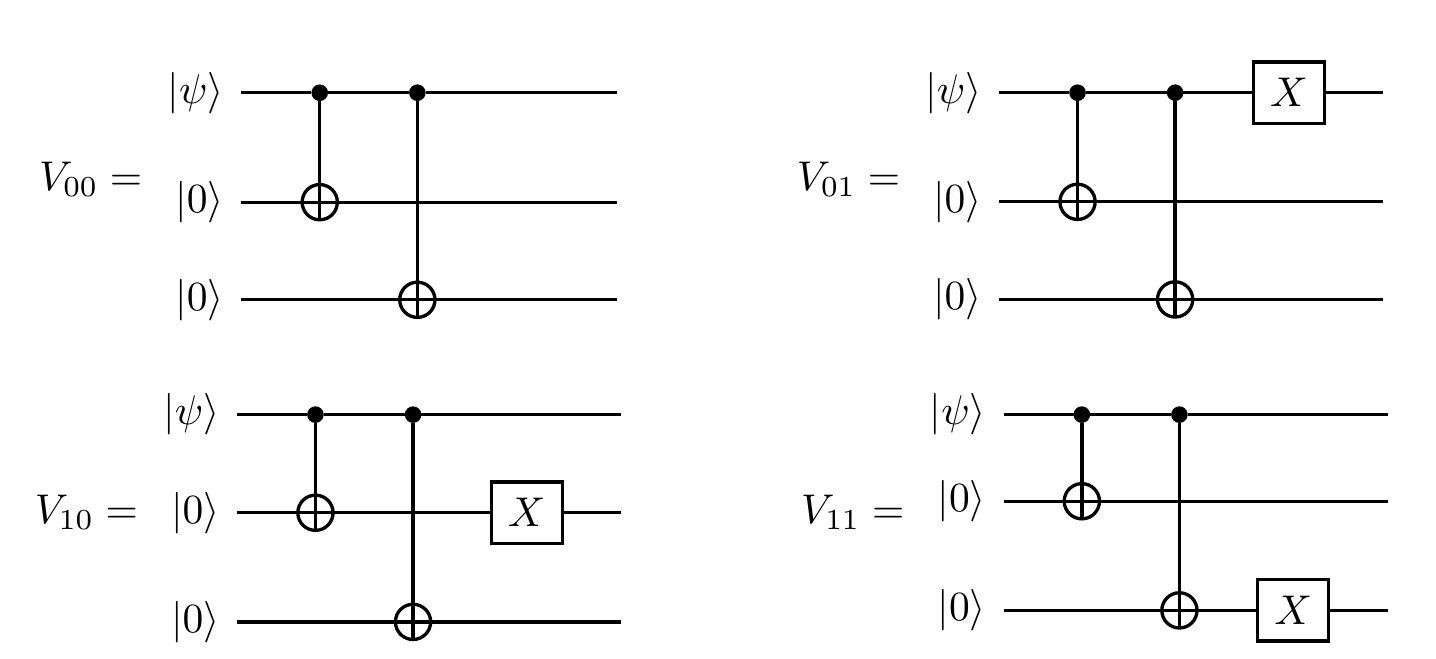## 13.5 Towards error correction

In Section 13.1, when Alice used a random choice of four isometries to produce a three-qubit output, notice how we can write \begin{aligned} V_{01} &= (\mathbf{1}\otimes\mathbf{1}\otimes X)V_{00} \\V_{01} &= (\mathbf{1}\otimes X\otimes\mathbf{1})V_{00} \\V_{01} &= (X\otimes\mathbf{1}\otimes\mathbf{1})V_{00} \end{aligned} and thus express all of the isometries in terms of V_{00}. In other words, rather than thinking of Alice as picking randomly between four different isometries, we can imagine that she always picks the encoding isometry V_{00}, and then some noisy process randomly applies one of the four errors \mathbf{1}, \mathbf{1}\mathbf{1}X, \mathbf{1}X\mathbf{1}, or X\mathbf{1}\mathbf{1}. Correcting the isometry then corresponds to identifying which error happened, fixing it, and then removing the encoding. This is the process of error correction in a nutshell.Figure 13.5: Quantum error correction can be thought of as a three-step process: encoding, transmitting through a noisy channel, and then detecting and decoding. We will give a more accurate depiction of an error correcting diagram, explaining what actually happens in the “detection & decoding portion” in Figure 13.8.

When we studied Pauli stabilisers in Section 7.2, we came across exactly the spaces of this example:The stabiliser formalism gives us a very natural way of describing the error correcting code, along with its correction:

• the codespace (i.e. the space with no error) is defined by the two stabilisers +ZZ\mathbf{1} and +\mathbf{1}ZZ
• the error can be determined by measuring the value of these two stabilisers; we simply have to find which of the four possible errors gives the correct (anti-)commutation relations as specified by the measurement outcomes \pm1.

Generalising this idea further is the subject of Section 13.6.

We reiterate that, with this formalism, not only can we correct errors such as X, but also those such as Pauli rotations e^{iX\theta} that act via |\psi\rangle \longmapsto \cos\theta|\psi\rangle + i\sin\theta X|\psi\rangle which, when we error correct, split into two cases: with probability \cos^2\theta we find that no error has happened; with probability \sin^2\theta we find that the error X has happened, and we correct it.264

1. See Exercise 13.11.3↩︎